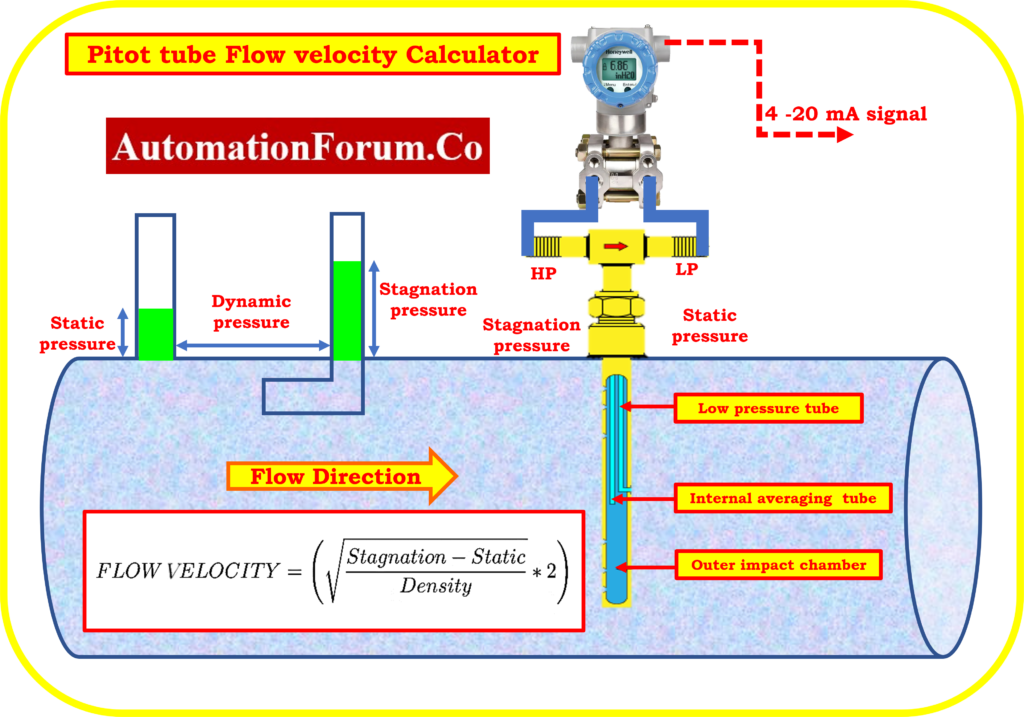# Pitot tube flow velocity calculator• One of the flow elements used to gauge the rate of flow in a pipeline, duct, etc. is the pitot tube. Pitot tubes are frequently utilised because of their inexpensiveness, convenience, and small size.
• The pitot tube’s construction is based on the measurement of the process’s static and stagnation pressure in the pipe.
• The difference between these two pressure readings indicates that dynamic pressure is just the point at which velocity becomes zero and pressure is converted.
•  By using this idea as a guide, the Pitot Tube flow calculator was created.
• When accuracy is minor, pitot tubes are typically employed in air ducts, flue gas paths, etc. to measure air speed.
• Additionally, the pitot tube will frequently become clogged with the duct particle present, requiring periodic purging of the tube.
• The high and low pressure sides of the above picture both include a hole wave passage for measuring pressure.
• The flowing medium’s stagnation pressure is detected by the upstream sensor, while the downstream sensor detects the static pressure.
• Both the pressure will serve as the second sensory element, similar to the transmitter’s diaphragm.
• You can calculate the dynamic pressure from the pressure differences to get the flowing medium’s velocity.
• The difference between static and stagnation pressure is shown on the right side of the diagram.
•  In order to measure the static pressure while the pipe is flowing, attach a clear, straight tube to the top. Even when the pressure condition varies, the static pressure will remain the same at any point along the pipe.
• However, if the standard Pitot Tube is inserted at the same time, the liquid level climbs above the static pressure level.
• The flow velocity decreases to zero as soon as it enters the Pitot Tube, which is the cause.
• The water level is raised above the level of static pressure by the velocity impact.
• The level above the steady pressure is known as the dynamic pressure. And when these pressures are added together, you have stagnation.

## Formula for calculation

The formula to calculate velocity using Bernoulli’s equals is provided below.

V is equal to sqrt (2*(static pressure minus stagnation pressure)/density).

To calculate the flow velocity (v), you need the values of static pressure (P), density (ρ), and stagnation pressure (P0). Rearranging the equation, we get:

v = sqrt((2 * (P0 – P)) / ρ)

Where:

P is the static pressure of the fluid

ρ (rho) represents the fluid density.

v is the flow velocity

P0 is the stagnation pressure

## Example calculation:

Given:

Static pressure, P = 1.2 MPa (megapascal)

Density, ρ = 1000 kg/m^3

Stagnation pressure, P0 = 1.4 MPa

Using the formula, we can calculate the flow velocity (v):

v = sqrt((2 * (1.4 MPa – 1.2 MPa)) / 1000 kg/m^3)

= sqrt((2 * 0.2 MPa) / 1000 kg/m^3)

= sqrt(0.4 MPa / 1000 kg/m^3)

= sqrt(0.0004)

v ≈ 0.020 m/s (approximately)

Therefore, the flow velocity in this example is approximately 0.020 m/s.

## Pitot tube flow velocity calculator

The ease of time is determined using the below Pitot Tube flow Calculator once all inputs have been entered.